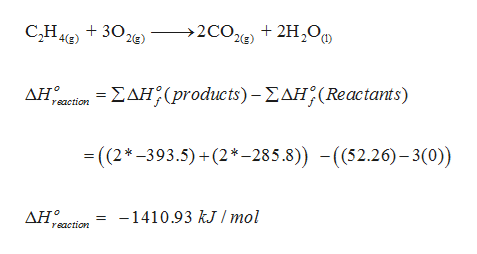# Calculate the heats of combustion for the following reactions. Use the standard enthalpies of formation. (a) C2H4(g) + 3O2(g) → 2CO2(g) + 2H2O(l)kJ/mol (b) 2H2S(g) + 3O2(g) → 2H2O(l) + 2SO2(g)kJ/mol

Question
2 views

Calculate the heats of combustion for the following reactions. Use the standard enthalpies of formation.

(a) C2H4(g) + 3O2(g) → 2CO2(g) + 2H2O(l)

kJ/mol

(b) 2H2S(g) + 3O2(g) → 2H2O(l) + 2SO2(g)

kJ/mol

check_circlehelp_outlineImage TranscriptioncloseC,H + 30e) →2CO% + 2H,O, 4(g) -ΣΔΗ(produw is) - ΣΔΗ; (Reactants) ΔΗ reaction = (2*-393.5) +(2*-285.8)) -(52.26)– 3(0)) -1410.93 kJ / mol ΔΗ reaction fullscreen

### Want to see the full answer?

See Solution

#### Want to see this answer and more?

Solutions are written by subject experts who are available 24/7. Questions are typically answered within 1 hour.*

See Solution
*Response times may vary by subject and question.
Tagged in

### Chemistry